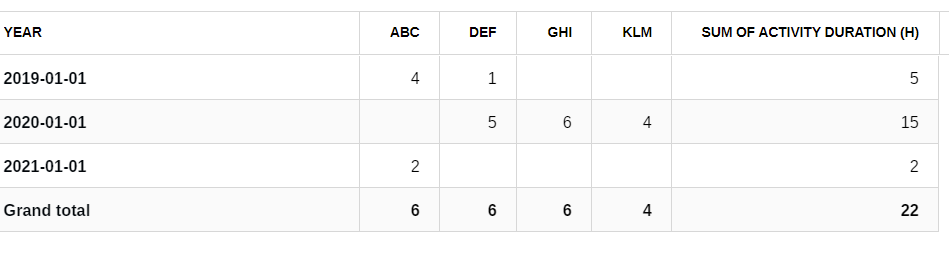# Calculation methods

### Calculation methods

Most charts allow you to select a calculation method. The 5 calculation methods are:

• Average: Average value of the selected field.
• Count: Number of records (rows).
• Count(unique): Number of records (rows) but removes duplicates (based on selected fields).
• Running total: Sum of the selected field (accumulated through time).
• Sum: Sum of the selected field.

### Examples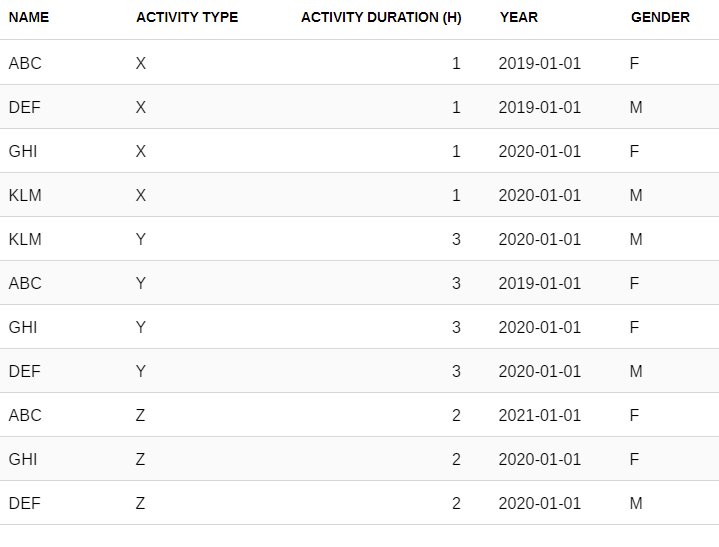With the example dataset presented above, you could do the following calculations:

 Average Average duration of activities per activity type Calculation= Average. Field= Duration. Rows= Activity type.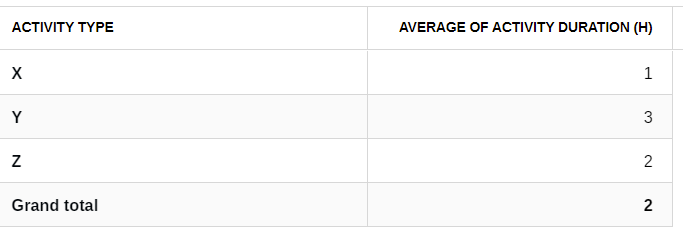Count Number of participations per year (if participants attend many activities, each activity/participant counts) Calculation=Count. Field=Name. Rows=year.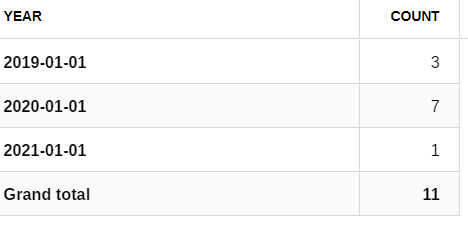Count(unique) Number of participations per year (if participants attend many activities, only 1 activity/participant counts) Calculation=Count(unique). Field=Name. Rows=year.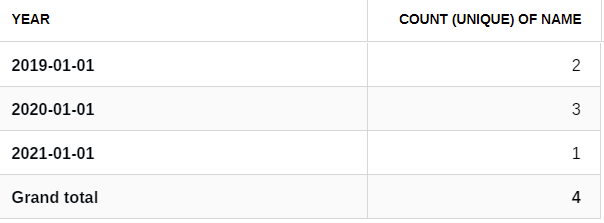Running total Total of hours participated per person by year (cumulated - and not just hours that were actually done during that period). Calculation= Running total. Field=Duration. Rows=Year. Columns=Name.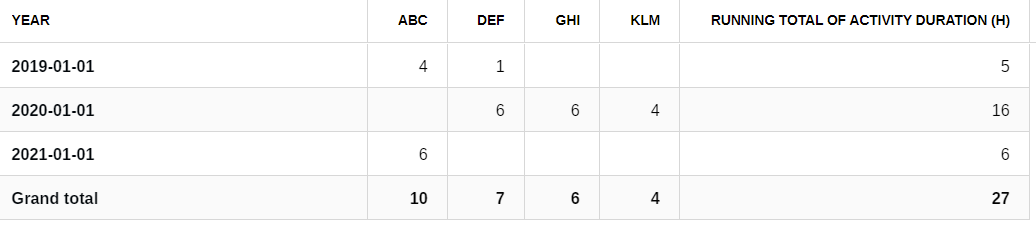Sum Total of hours participated per person per year. Calculation= Sum. Field=Duration. Rows=Year. Columns=Name.